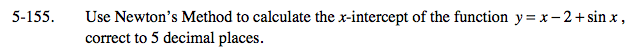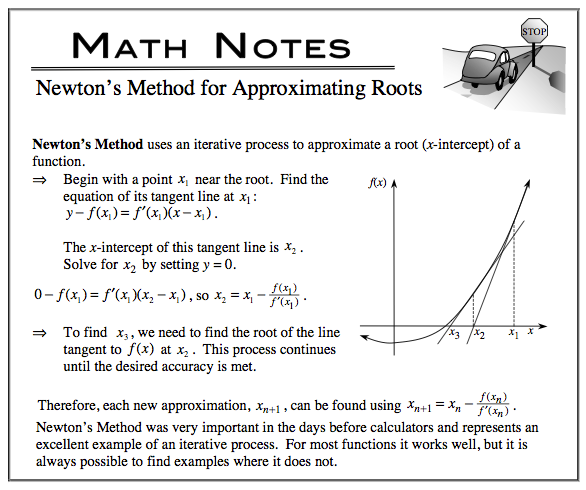### Home > CALC > Chapter 5 > Lesson 5.5.1 > Problem5-155

5-155.

Use Newton's Method to calculate the x-intercept of the function y = x − 2 + sin x, correct to 5 decimal places. Homework Help ✎A reasonable estimate is x1 = 1.

$y(1)=1-2+\sin(1)\approx–0.1585$